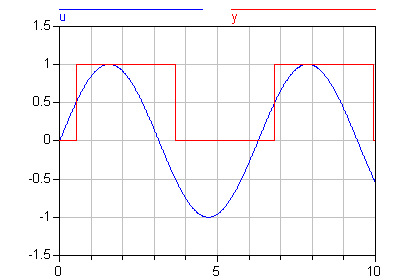# 'pre()'

pre()# Information

This information is part of the Modelica Standard Library maintained by the Modelica Association.

Refer to left limit

`pre(y)`

#### Description

Returns the "left limit" y(tpre) of variable y(t) at a time instant t. At an event instant, y(tpre) is the value of y after the last event iteration at time instant t. The pre operator can be applied if the following three conditions are fulfilled simultaneously:

• variable y is a subtype of a simple type
• y is a discrete-time expression
• the operator is not applied in a function class

The first value of pre(y) is determined in the initialization phase.

A new event is triggered if at least for one variable v "pre(v) <> v" after the active model equations are evaluated at an event instant. In this case the model is at once reevaluated. This evaluation sequence is called "event iteration". The integration is restarted, if for all v used in pre-operators the following condition holds: "pre(v) == v".

[If v and pre(v) are only used in when clauses, the translator might mask event iteration for variable v since v cannot change during event iteration. It is a "quality of implementation" to find the minimal loops for event iteration, i.e., not all parts of the model need to be reevaluated.

The language allows mixed algebraic systems of equations where the unknown variables are of type Real, Integer, Boolean, or an enumeration. These systems of equations can be solved by a global fix point iteration scheme, similarly to the event iteration, by fixing the Boolean, Integer, and/or enumeration unknowns during one iteration. Again, it is a quality of implementation to solve these systems more efficiently, e.g., by applying the fix point iteration scheme to a subset of the model equations.]

Note that pre(v) requires the argument to be a variable and a discrete-time expression, this formulation was chosen to allow using pre(v) both for discrete-time variables and for continuous-time variables inside when-clauses.

#### Examples

```model Hysteresis
Real u;
Boolean y;
equation
u = Modelica.Math.sin(time);
y = u > 0.5 or pre(y) and u >= -0.5;
end Hysteresis;```